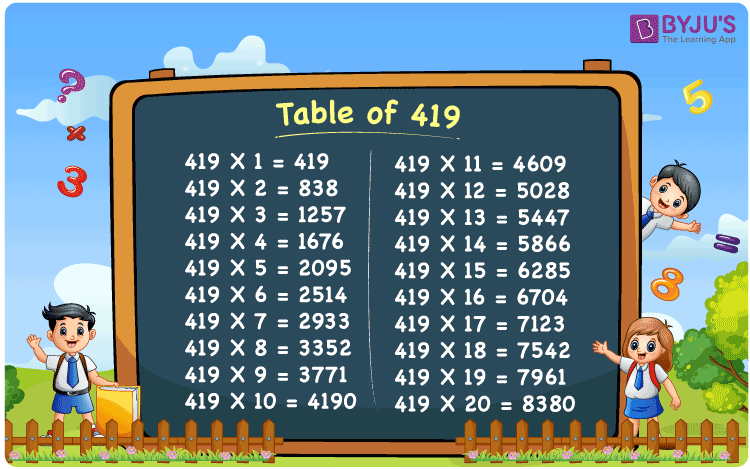Checkout JEE MAINS 2022 Question Paper Analysis : Checkout JEE MAINS 2022 Question Paper Analysis :

# Table of 419

The table of 419 is given here to help the students learn the multiplication of 419 through 20 times. This will help them solve problems involving the multiples of 419 effortlessly. As we know, multiplication tables have huge applications in many areas of mathematics. We also apply these maths tables to compute multiplication-related numbers in our daily lives.

## Table of 419 Chart## What is the 419 Times Table?

The 419 times table is given below in terms of both addition and multiplication to help the students memorise the table of 419 quickly.

 Using multiplication Using repeated addition 419 × 1 = 419 419 419 × 2 = 838 419 + 419 = 838 419 × 3 = 1257 419 + 419 + 419 = 1257 419 × 4 = 1676 419 + 419 + 419 + 419 = 1676 419 × 5 = 2095 419 + 419 + 419 + 419 + 419 = 2095 419 × 6 = 2514 419 + 419 + 419 + 419 + 419 + 419 = 2514 419 × 7 = 2933 419 + 419 + 419 + 419 + 419 + 419 + 419 = 2933 419 × 8 = 3352 419 + 419 + 419 + 419 + 419 + 419 + 419 + 419 = 3352 419 × 9 = 3771 419 + 419 + 419 + 419 + 419 + 419 + 419 + 419 + 419 = 3771 419 × 10 = 4190 419 + 419 + 419 + 419 + 419 + 419 + 419 + 419 + 419 + 419 = 4190

## Multiplication Table of 419

The multiplication table of 419 is given here, which will help you to learn the table of 419 up to 20 times.

 419 × 1 = 419 419 × 2 = 838 419 × 3 = 1257 419 × 4 = 1676 419 × 5 = 2095 419 × 6 = 2514 419 × 7 = 2933 419 × 8 = 3352 419 × 9 = 3771 419 × 10 = 4190 419 × 11 = 4609 419 × 12 = 5028 419 × 13 = 5447 419 × 14 = 5866 419 × 15 = 6285 419 × 16 = 6704 419 × 17 = 7123 419 × 18 = 7542 419 × 19 = 7961 419 × 20 = 8380

## Solved Example

Question:

The price of a handicraft is Rs. 419. Find the amount to be paid for 15 such handicrafts.

Solution:

Given,

Price of 1 handicraft = Rs. 419

Number of handicrafts = 15

Total amount = ?

Using the table of 419, 419 × 15 = 6285

Amount to be paid for 15 handicrafts = Rs. 419 × 15 = Rs. 6285.

## Frequently Asked Questions on Table of 419

### What is the table of 419?

The table of 419 can be written by multiplying 419 by numbers 1, 2, 3, and so on. Below are some of the results of the multiplication table of 419.
419 × 1 = 419
419 × 2 = 838
419 × 3 = 1257
419 × 4 = 1676
419 × 5 = 2095

### Why do we learn the table of 419?

Learning the table of 419 will help you to solve the numerical simplifications quickly with accuracy.

### How many times of 419 is 4609?

11 times of 419 or 419 times 11 is 4609, i.e. 419 × 11 = 4609.